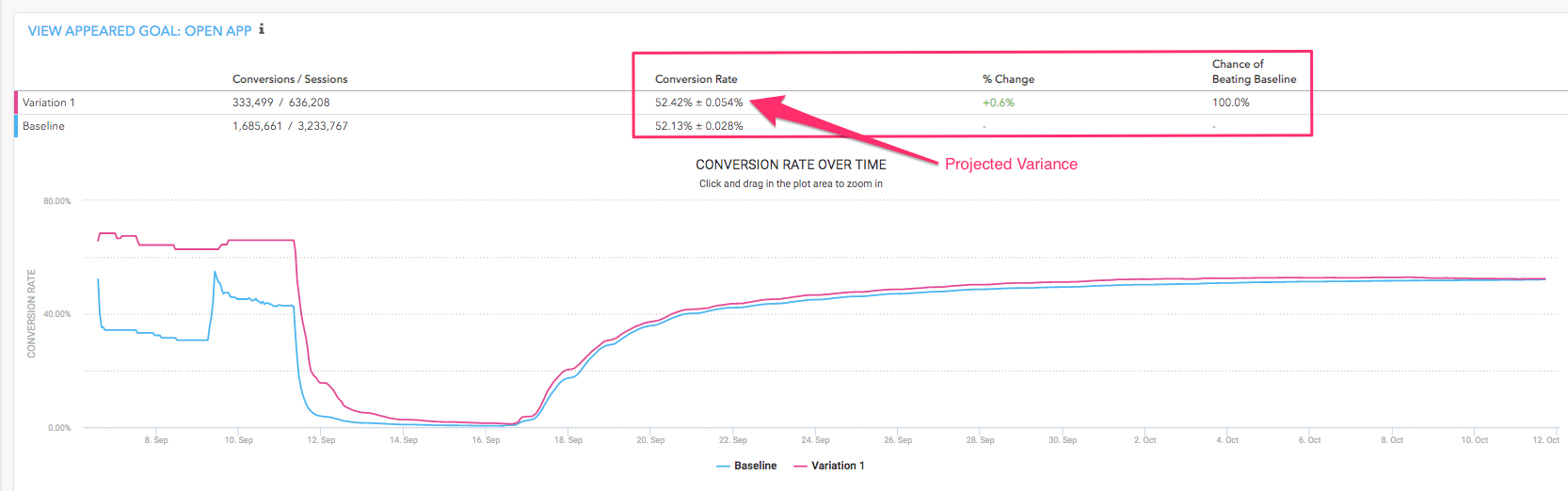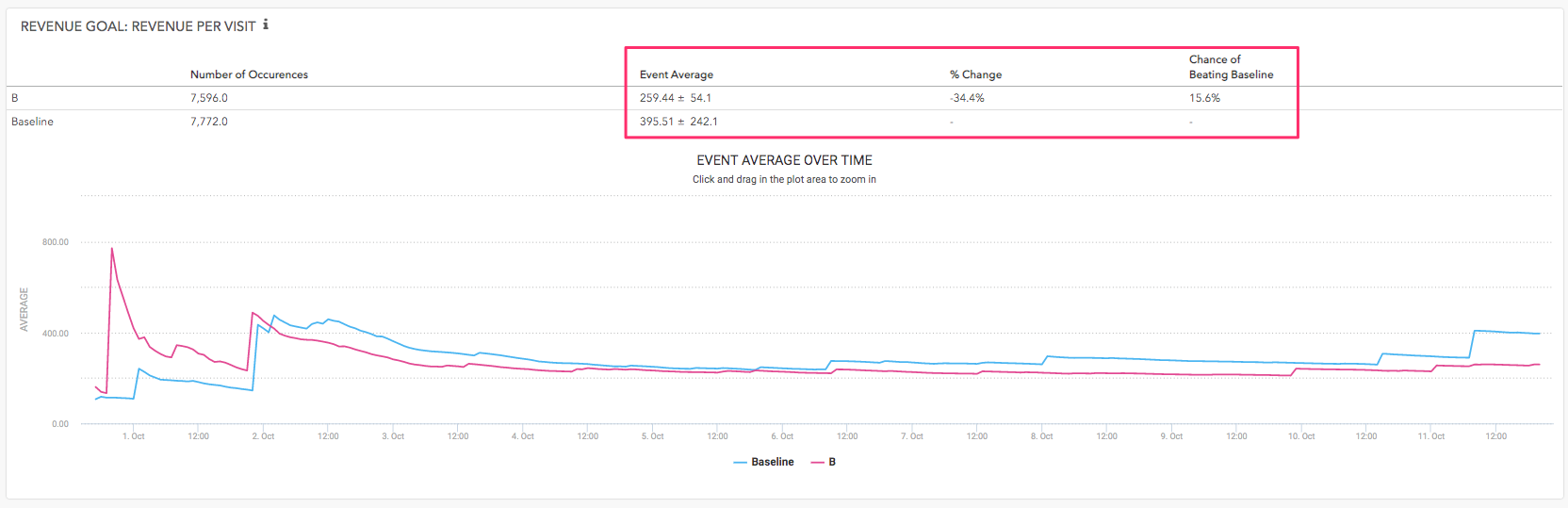# How Experiment Statistics are Calculated

Taplytics uses two methods to calculate the effectiveness of goals created to evaluate your experiments:

• Z-Score - is used for binary conversion goals i.e. button clicks / app sessions goals

• Two-Tailed T-Test - is used for value optimization goals where the comparison is the difference between two average values

Both methods test for a 95% confidence level.

Below is a brief definition the calculations use to determine the success of each type of goal when viewing the Experiment Results page on the Taplytics Dashboard:

# Binary Conversion GoalsConversion Rate
This is a straight calculation of the number of conversion events divided by the number of events you've chosen to be the denominator, most commonly this is app session.

% Change
This is calculated in the following way:

(Conversion Rate Variation - Conversion Rate Baseline) / Conversion Rate Baseline

Projected Variance
This is calculated using standard methods for z-scores.

Chance of Beating Baseline
This number will fluctuate as your experiment accumulates more data.

Once the Chance of Beating Baseline reaches 100% it confirms that the variation has reached a 95% confidence between Baseline and Variation.

# Value Optimization GoalsEvent Average
Is the calculated mean of the event average.

Percentage Change
This number is calculated below:

(Event Average Variation - Event Average Baseline) / Event Average Baseline

Chance of Beating Baseline
Similar to above - this number will fluctuate as your experiment accumulates more data.

Once the Chance of Beating Baseline reaches 100% it confirms that the variation has reached a 95% confidence between Baseline and Variation.